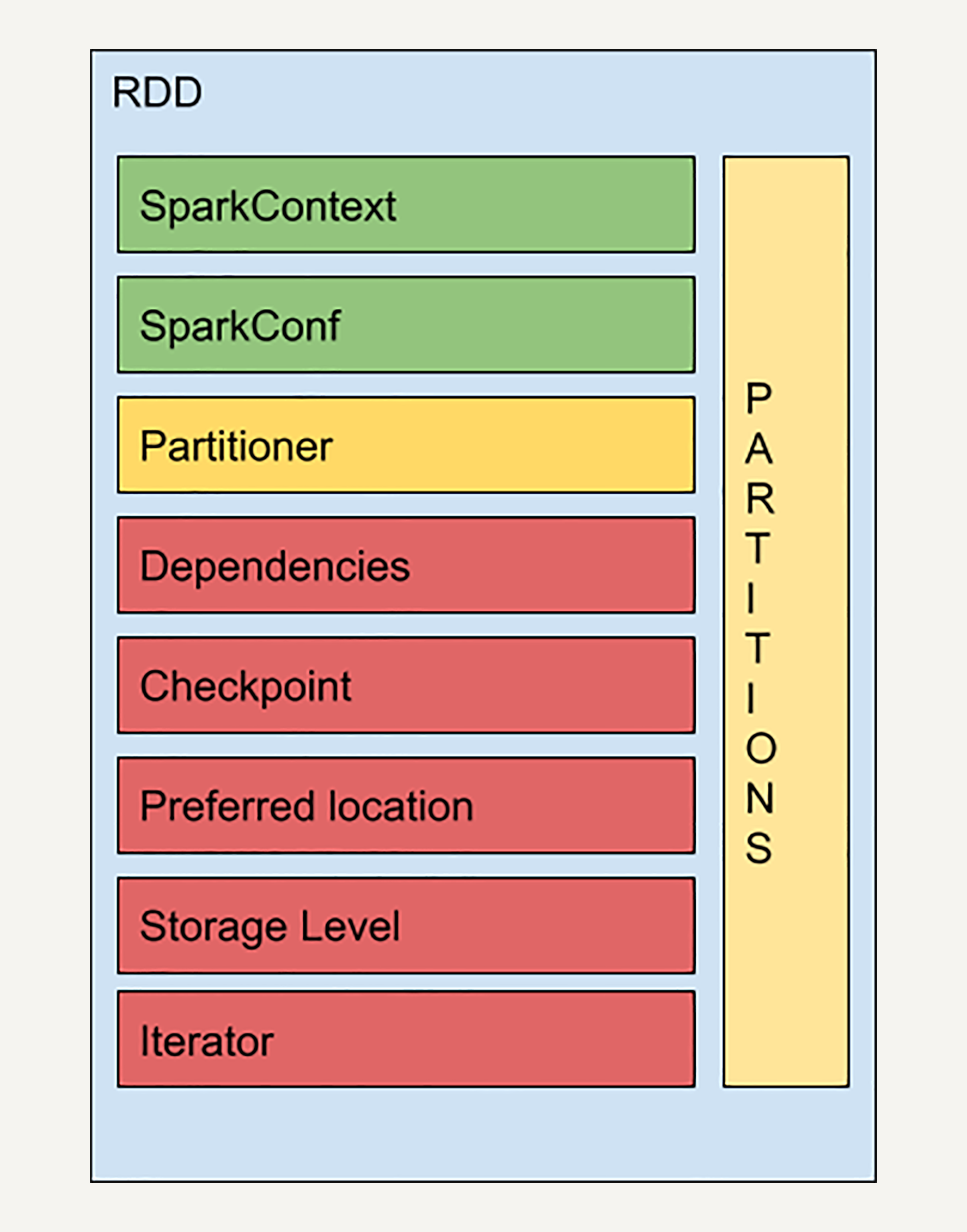# Spark

## 大数据基础

Posted by Galvin on August 12, 2019

### Spark

• Spark基本的数据结构：弹性分布式数据集（Resilient Distributed Dataset，RDD），他代表一个可被分区的只读数据集

### RDD

#### 分区（Partition）

• 同一个RDD包含的数据被存储在系统的不同节点中
• RDD只是抽象意义的数据集合，分区内部并不存储具体的数据。每个分区指向一个存储在内存或者硬盘中的数据块（Block）
• RDD中每个分区存有它在该RDD中的index。通过RDD的ID和分区index可以唯一确定对应数据块的编号

#### 不可变性

• 每个RDD都是只读的，它所包含的分区信息不可以被改变

#### 并行操作

• 单个RDD的分区特性，使得它天然支持并行操作，不同节点的数据可以被分别处理，产生一个新的RDD

### RDD结构### RDD数据操作

#### 转化(Transformation)

• Map
``````rdd = sc.parallelize(["b", "a", "c"])
rdd2 = rdd.map(lambda x: (x, 1)) // [('b', 1), ('a', 1), ('c', 1)]

``````
• Filter
``````rdd = sc.parallelize([1, 2, 3, 4, 5])
rdd2 = rdd.filter(lambda x: x % 2 == 0) // [2, 4]
``````
• mapPartitions(输入是应用于RDD的每个分区)
``````rdd = sc.parallelize([1, 2, 3, 4], 2)
def f(iterator): yield sum(iterator)
rdd2 = rdd.mapPartitions(f) // [3, 7]
``````
• groupByKey
``````rdd = sc.parallelize([("a", 1), ("b", 1), ("a", 2)])
rdd.groupByKey().collect()
//"a" [1, 2]
//"b" 
``````

#### 动作（Action）

• Collect
``````rdd = sc.parallelize(["b", "a", "c"])
rdd.map(lambda x: (x, 1)).collect() // [('b', 1), ('a', 1), ('c', 1)]
``````
• Reduce
``````from operator import add
sc.parallelize([1, 2, 3, 4, 5]).reduce(add)  // 15
``````
• CountByKey
``````rdd = sc.parallelize([("a", 1), ("b", 1), ("a", 1)])
sorted(rdd.countByKey().items()) // [('a', 2), ('b', 1)]
``````

### 总结

Spark 在每次转换操作的时候使用了新产生的 RDD 来记录计算逻辑，这样就把作用在 RDD 上的所有计算逻辑串起来形成了一个链条，但是并不会真的去计算结果。当对 RDD 进行动作 Action 时， Spark 会从计算链的最后一个 RDD 开始，利用迭代函数 （Iterator） 和计算函数（Compute），依次从上一个 RDD 获取数据并执行计算逻辑，最后输出结果。